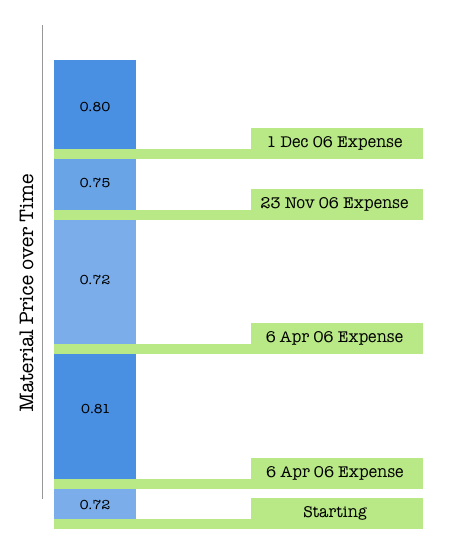# How are unit costs for materials calculated?

There are two separate costs calculated for each of your materials: Unit Cost and Actual Unit Cost. The essential difference between the two is that your Actual Unit Cost factors in shipping and discounts, where your Unit Cost is the base price for the material purchased.

Both are calculated in your nominated Tracking Unit and are calculated using the Moving Weighted Average Cost Method. This method is an IRS approved method of calculating inventory that uses a moving average calculation of all stock changes made (via Material Inventory Adjustments) to determine the exact value of your stock on any particular day based on the current "pool" of your stock available.

Each time you create a new expense for a material, all available stock is recalculated as a single "pool" to include the newly added quantities and their actual unit costs. When a manufacture is recorded and stock is decreased, the available stock is again recalculated based on the materials that are left using the same moving cost method.

The diagram below shows a visualisation of how unit costs change over time in Craftybase, based on expenses occurring with different average unit costs. The material starts with a starting unit cost of 0.72, however expenses over time slowly increase and decrease the unit cost of the material (from 0.72 up to 0.81). These costs can be pin-pointed at any time between the expenses using the moving average cost method.